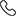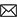Sales :9214233303 / Tech. Support Desk :9214233360/40 (9AM ~ 6PM)# Remembering formulas for JEE: Best Guide For JEE Aspirants

27 Sep, 2022, By Tanvi SharmaAlong with the high level of competition, what makes the JEE exam the most backbreaking one? Well, it's the broad syllabus. The vastness of the JEE syllabus makes it a strenuous task to remember everything at your fingertips. The students appearing for the JEE exam find it a complex task to remember all the formulas, concepts, topics, theories, etc to the core. But among these, where most students get stuck is the formula part, which forms the most part of the JEE exam.

So, if you are finding it difficult to learn all the formulas for JEE then in this article you can find some very beneficial tips to deal with the problem.

## Simple yet effective tips to remember formulas for JEE

Here are the simple yet effective tips to remember formulas for JEE:

### 1. Reading and remembering make memorizing easy

When it comes to learning the formulas by heart, reading and remembering are the two important things you need to keep up with, to make the task of learning formulas for JEE easy. The questions asked in the JEE exam contain a maximum percent of formula-based questions and hence it is mandatory to memorize the formulas. Glancing through the derivations will help you understand the source of formulas.

Know about: JEE 2022 College Predictor

You can memorize the formulas by reading them, revising them, and writing them on a daily basis, this will help you in learning the formulas the easy way. Memorizing formulas on the same day of examination is not only hard but also foolish and thus it is suggested to start learning the formulae the moment you begin your preparation.

### 2. Focusing on the core concepts

The topics of the JEE exam need an in-depth understanding of the topics. If you want to crack the JEE exam then cramming won't do the trick. Having a good understanding of all the subjects: Physics, Chemistry, and Math through real-life examples will help you grasp the formulas for JEE in a better way.

### 3. Practice yield perfections

Now after you have penned down all the important formulae and have memorized them to perfection it is time to start implementing these formulae by giving in more and more practice. You are required to solve a plethora of problems if you want to ace the JEE exam. Even after reading so much, if you are not spending enough time practicing, you are going to face problems in solving, especially in mathematics.

To leave other students behind you need to develop your problem-solving skills that can only be achieved by practicing. Learning the formulae of JEE well and putting them into practice will help you develop shortcuts to more complex questions.

### 4. Visualizing the formulas

You must be thinking how visualizing formulas is of any help but to your wonder, it is more important than you can ever imagine. While memorizing, try to visualize the formulas and their symbols as well as derivations. Note the formulas down on sticky notes and paste them where you can have easy visual access.

Also Read: JEE Main Previous Year Question Paper with Solutions

### 5. Maintain a formula book

To ease your learning of formulas for JEE easily, you need to start maintaining a book that includes all the formulas for all three subjects. You divide the books into three sections: Physics, Chemistry, and Math and you can jot down the formulas of the respective subject in the section of the concerned section. Having the formulas written in the same books will provide you ease in memorizing them and revising and having a glance at them all together.

### 6. Test the formulas regularly

It is not just the problem questions that you should keep the regular practice on but also the formulas. Keep testing the formulas to check whether you are having problems remembering them. This will give you a quick self-analysis of how well you remember the formulas.

### 7. Say no to stress

The exam incorporates a vast JEE syllabus and to be able to crack this exam it is mandatory to have the entire syllabus at your fingertips, chiefly the formulas. And stressing out is what the students resort to at first. But smartness lies in approaching the formulae, concepts, etc with a relaxed mind. Studying with a stressed mind will only create more confusion. Hence studying smart and learning better is a crucial part of your preparation journey and thus it needs to be done with a calm and cool mind. And only then will you be able to crack the JEE exam.

It is a fact that smart work triumphs over hard work. If you are putting in all your efforts to ace the JEE exam and still missing the excellence then it is just about time that you start putting in the effort where it is required.

In the initial stages of your JEE preparation memorizing the formula for JEE can be a bit boring but after you will acquaint yourself with the same you will find it quite easy.

Mostly numerical-based questions are asked in the JEE exam and to be able to solve these questions like a topper it is predominant to memorize the formula of JEE well. And following these simple yet easy tips to learn the formulas for JEE, this task will be quite undemanding.

#### What things should I do and don't do in preparation for the NEET exam?

Given are some of the mistakes that you should avoid while preparing for the NEET exam:

• Not following a timetable

• Not concentrating on the Speed

• Being Overconfident About the Preparation

• Neglecting Health

#### What are the books you followed for the NEET?

To prepare for the NEET 2023 exam then it is important that you take help from the right books. If you are looking for the right study material then you can opt for the best courses provided by ETOOS India along with the brilliant courses, it provides well-structured study material curated by a panel of expert teachers. The study material is formulated in a way to make learning easy for you.

#### How do I maintain consistency for the NEET to score 700+?

The given tips will help you score 700 marks in the NEET 2023:

• Prepare a study plan

• Practice will make you perfect